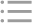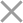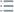Pour obtenir une clé
d'essai remplissez le formulaire ci-dessous
Team licenсe
Enterprise licence
** En cliquant sur ce bouton, vous acceptez notre politique de confidentialité.Demandez des tariffs
Nouvelle licence
Renouvellement de licence
--Sélectionnez la devise--
USD
EUR
RUB
* En cliquant sur ce bouton, vous acceptez notre politique de confidentialitéFree PVS-Studio license for Microsoft MVP specialists
** En cliquant sur ce bouton, vous acceptez notre politique de confidentialité.To get the licence for your open-source project, please fill out this form
** En cliquant sur ce bouton, vous acceptez notre politique de confidentialité.I am interested to try it on the platforms:
 Windows Linux macOS PVS-Studio for .NET Core JetBrains Rider
** En cliquant sur ce bouton, vous acceptez notre politique de confidentialité.Votre message a été envoyé.

Nous vous répondrons à

Si vous n'avez toujours pas reçu de réponse, vérifiez votre dossier
Spam/Junk et cliquez sur le bouton "Not Spam".
De cette façon, vous ne manquerez la réponse de notre équipe.

>
>
>
V1085. Negative value is implicitly con…Introduction
Analyzing projects
On Windows
On Linux and macOS
Continuous use of the analyzer in software development
Deploying the analyzer in cloud Continuous Integration services
Managing analysis results
Additional configuration and resolving issues
Analyzer diagnostics
General Analysis (C++)
General Analysis (C#)
General Analysis (Java)
Diagnosis of micro-optimizations (C++)
Diagnosis of 64-bit errors (Viva64, C++)
Customer specific requests (C++)
MISRA errors
AUTOSAR errors
OWASP errors (C++)
OWASP errors (C#)
OWASP errors (Java)
Problems related to code analyzerContents

# V1085. Negative value is implicitly converted to unsigned integer type in arithmetic expression.

20 Mai 2022

The analyzer has detected a negative value converted to an unsigned type in an arithmetic expression. According to implicit conversion rules in C/C++, a signed integer that has the same size as an unsigned one turns into an unsigned one. When a negative number is converted to an unsigned type, it is wrapped modulo '(2 ^ n) + 1', where n is the number of bits in the integer. This situation does not lead to undefined behavior, but it can provoke unexpected results.

Consider an example:

``````void foo()
{
char *p = (char *) 64;
int32_t a = -8;
uint32_t b = 8;
p = p + a * b;
}``````

On a 32-bit system, the pointer is 0x0. On 64-bit, it is 0x0000'0001'0000'0000. This may be unexpected for a programmer. Let's figure out why this is happening.

The 'a' variable has the signed type 'int32_t'. This means that its size is 4 bytes, and it can receive values in the range from -2'147'483'648 to 2'147'483'647. The 'b' variable has the 'uint32_t' type. It also has a size of 4 bytes but, unlike the 'a' variable, it can take values in the range from 0 to 4'294'967'295. This happens because the highest bit in the signed integer is preserved for the sign. Because of this limitation, the maximum value of a signed integer is half of the value of an unsigned one.

According to C++ language rules, if operands have types with the same rank in a binary operation and one of the operands has a signed type while the other is unsigned, the operand that has a signed type is implicitly converted to unsigned.

In the 'a * b' expression, the operand types ('int32_t' and 'uint32_t') have the same rank. Therefore, the 'a' operand that stores the '-8' value is implicitly converted to an unsigned type 'uint32_t'. As a result of this conversion, its value becomes 4'294'967'288. Next, it is multiplied by the 'b' variable that stores the '8' value. The result obtained is 34'359'738'304. It is outside the range of the 'uint32_t' type variable. It will be wrapped modulo '2 ^ 32'. Thus, the result of the 'a * b' expression will be 34'359'738'304 % 4'294'967'296 = 4'294'967'232.

The addition operator 'p + a * b' has the following operand types: 'char *' and 'uint32_t', respectively. According to the C++ standard, the resulting type is 'char *', and the result is the sum of the left and right operands. When 64 and 4'294'967'232 are added, the result is 4'294'967'296.

On a 32-bit platform, the pointer size is 4 bytes. Therefore, its maximum value is 4'294'967'295. Since 4'294'967'296 is greater, the result is wrapped modulo '2 ^ 32' as in the previous addition operation. The result will be 4'294'967'296 % 4'294'967'296 = 0. So, 'p + a * b' equals zero.

On a 64-bit platform, the pointer size is 8 bytes. And unlike a 32-bit platform, its maximum value is much greater than 4'294'967'296. Since there will be no wrapping, the result of 'p + a * b' is 4'294'967'296 in the decimal system or 0x0000'0001'0000'0000 in the hexadecimal system.

We can fix the above example by using signed types:

``````void foo()
{
char *p = (char *) 64;
int32_t a = -8;
uint32_t b = 8;
p = p + a * static_cast<int32_t>(b);
}``````

Not all signed types conversions to unsigned will trigger the diagnostic. It will issue a warning only for expressions whose result will be different from the result obtained when only signed types are used. Consider an example:

``````void foo()
{
unsigned num = 1;
unsigned res1 = num + (-1); // ok
unsigned res5 = num + (-2); //+V1085

unsigned res2 = num - (-1); // ok

unsigned res3 = num * (-1); //+V1085

unsigned res4 = num / (-1); //+V1085
unsigned res6 = num / (-2); // ok

unsigned num2 = 2;

unsigned res7 = num2 / (-1); //+V1085
}``````

Lines containing the 'ok' comment will not trigger the V1085 warning. Here are the results of each expression with signed and unsigned variants:

``````num  +   (signed)(-1) => 1 + (-1) => 0
num  + (unsigned)(-1) => 1 + 4294967295 = 0

num  +   (signed)(-2) => 1 + (-2) => -1
num  + (unsigned)(-2) => 1 + 4294967294 = 4294967295

num  -   (signed)(-1) => 1 – (-1) => 2
num  - (unsigned)(-1) => 1 – (4294967295) => 2

num  *   (signed)(-1) => 1 * (-1) => -1
num  * (unsigned)(-1) => 1 * (4294967295) => 4294967295

num  /   (signed)(-1) => 1 / (-1) => -1
num  / (unsigned)(-1) => 1 / 4294967295 => 0

num  /   (signed)(-2) => 1 / (-2) => 0
num  / (unsigned)(-2) => 1 / 4294967294 => 0

num2 /   (signed)(-2) => 2 / (-2) => -1
num2 / (unsigned)(-2) => 2 / 4294967294 => 0``````

The analyzer will not issue a warning in places where the results match.

Note. The considered problems overlap with the topic of porting applications from 32-bit to 64-bit systems. See the following article: "A collection of examples of 64-bit errors in real programs."

This diagnostic is classified as:

 CWE-190CERT-INT32-CNous utilisons des cookies pour améliorer votre expérience de navigation. En savoir plus